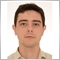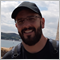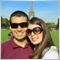# Issue when BackTesting with CopyTicksRange & CopyTicks in EA1023

Hello there!

I'm trying to get ticks for some calc with CopyTicksRange function in EA (please,see code below). It works fine in real account, give the proper results. But when backtesting, it doesn't work. I'm currently working in Brazilian market with asset WING19. As I could understand so far, the issue may be on dates, as it reflects the total sec between desired date and D'01.01.1970 00:00:00'.

Trying to eliminate possible problems with CopyTicksRange, I also implemented CopyTicks function, both are working fine in real account, not in backtesting.

Also, I couldn't understand the reason why in real account it gives me the true asset historical begin, while in backtesting it gives a year back as asset historical begin.

```//+------------------------------------------------------------------+
//|                                                       Ajuste.mq5 |
//|                        Copyright 2018, MetaQuotes Software Corp. |
//|                                             https://www.mql5.com |
//+------------------------------------------------------------------+
#property version   "1.00"

//+------------------------------------------------------------------+
//| CÁLCULO DO AJUSTE DE PREÇOS BMF                                  |
//+------------------------------------------------------------------+
double CarregaArrayTicksAjuste(datetime TDATAHORAINICIAL)
{

MqlDateTime today;
MqlTick TickAjuste[];

double Ajuste=0;
double totalvol=0;
TimeToStruct(TDATAHORAINICIAL,today);

today.hour=15;
today.min=50;
today.sec=0;

datetime inicio=StructToTime(today);
today.hour=16;
today.min=00;
today.sec=0;
datetime fim=StructToTime(today);

if(StringSubstr(_Symbol,0,2)=="WI")
{

today.hour=17;
today.min=20;
today.sec=0;

inicio=StructToTime(today);
today.hour=17;
today.min=35;
today.sec=0;
fim=StructToTime(today);

}
else
if(StringSubstr(_Symbol,0,2)=="WD")
{

today.hour=15;
today.min=50;
today.sec=0;

inicio=StructToTime(today);
today.hour=16;
today.min=00;
today.sec=0;
fim=StructToTime(today);

}
int Candles=Bars(_Symbol,PERIOD_M1,inicio,fim);
int Retorno=Bars(_Symbol,PERIOD_M1,fim,TimeCurrent())-1;
long Ticks=0;
MqlRates mrate[];
int copiou=CopyRates(_Symbol,PERIOD_M1,Retorno,Candles,mrate);
int barras=0;

for(int z=0;z<Candles-1;z++)
{
Ticks=Ticks+mrate[z].tick_volume;
barras=barras+1;
}

Print("Início é ",inicio," Término é ", fim);

ArrayFree(TickAjuste);
{
for(int i=0;i<ArraySize(TickAjuste);i++)
{
Ajuste=Ajuste+(TickAjuste[i].last*TickAjuste[i].volume);
totalvol=totalvol+TickAjuste[i].volume;
}

if(totalvol<1) totalvol=1;
Ajuste=NormalizeDouble(Ajuste/totalvol,0);

return Ajuste;
} else return -1;
}

//+------------------------------------------------------------------+
//| Expert initialization function                                   |
//+------------------------------------------------------------------+
int OnInit()
{
return(INIT_SUCCEEDED);
}
//+------------------------------------------------------------------+
//| Expert tick function                                             |
//+------------------------------------------------------------------+
void OnTick()
{
//--- Definições diversas de tempo, barras, etc
datetime FCandleT=StringToTime(TimeToString(iTime(_Symbol,PERIOD_D1,1),TIME_DATE) + " 00:00:00");
datetime LCandleT=StringToTime(TimeToString(iTime(_Symbol,PERIOD_D1,1),TIME_DATE) + " 23:59:59");
int TDBars=Bars(_Symbol,PERIOD_M15,FCandleT,LCandleT);
int Barras=Bars(_Symbol,PERIOD_M15,StringToTime(TimeToString(TimeCurrent(),TIME_DATE) + " 00:00:00"),TimeCurrent());
static datetime Ontem;

if(TDBars>=1 && Ontem!=FCandleT)
{
Ontem=FCandleT;
//--- To be used to store the prices, volumes and spread of each bar
MqlRates mrate[];
int copiou=CopyRates(_Symbol,PERIOD_M15,Barras,TDBars,mrate);
datetime Ultimo=mrate[TDBars-1].time;
datetime Primeiro=mrate.time;

double Ajuste=SymbolInfoDouble(_Symbol, SYMBOL_SESSION_CLOSE);
Print("Ajuste INFO do último pregão é: ",Ajuste);
}
}
//+------------------------------------------------------------------+
```40861

Paulo Garcia:

Hello there!

I'm trying to get ticks for some calc with CopyTicksRange function in EA (please,see code below). It works fine in real account, give the proper results. But when backtesting, it doesn't work. I'm currently working in Brazilian market with asset WING19. As I could understand so far, the issue may be on dates, as it reflects the total sec between desired date and D'01.01.1970 00:00:00'.

Trying to eliminate possible problems with CopyTicksRange, I also implemented CopyTicks function, both are working fine in real account, not in backtesting.

Also, I couldn't understand the reason why in real account it gives me the true asset historical begin, while in backtesting it gives a year back as asset historical begin.

What that means "doesn't work" ? What are the results ?296

I'm facing same problem, also trading US dolar and stock index in Brazilian market. I use the structure below, and it works perfectly during the day (besides it's an ugly solution), but I can't backtest (it simply gives me incorrect numbers). I'm sure it's something related to CopyTicks. Anyone can help?

void OnTick()

{

CopyTicks ( ref_Symbol, tick_array, COPY_TICKS_TRADE, 0, 10);

for(int i=0;i<10;i++)

{

tick = tick_array [i];

neg_time = tick.time_msc;

if(tick.flags == 56 && tick.volume_real >= VolumeTriggerInput && TrendingTimeCheck && neg_time > temp_neg_time) //BUY CASE

{Multiplier = tick.volume_real / VolumeTriggerInput; temp_neg_time = tick.time_msc; MarketBuy();}

if(tick.flags == 88 && tick.volume_real >= VolumeTriggerInput && TrendingTimeCheck && neg_time > temp_neg_time) //SELL CASE

{Multiplier = tick.volume_real / VolumeTriggerInput; temp_neg_time = tick.time_msc; MarketSell();}

}

ZeroMemory(tick_array);

}Moderator
26722

Paulo Possobon:

use the code button (Alt+S) when pasting code257

Paulo Possobon:

I'm facing same problem, also trading US dolar and stock index in Brazilian market. I use the structure below, and it works perfectly during the day (besides it's an ugly solution), but I can't backtest (it simply gives me incorrect numbers). I'm sure it's something related to CopyTicks. Anyone can help?

void OnTick()

{

CopyTicks ( ref_Symbol, tick_array, COPY_TICKS_TRADE, 0, 10);

for(int i=0;i<10;i++)

{

tick = tick_array [i];

neg_time = tick.time_msc;

if(tick.flags == 56 && tick.volume_real >= VolumeTriggerInput && TrendingTimeCheck && neg_time > temp_neg_time) //BUY CASE

{Multiplier = tick.volume_real / VolumeTriggerInput; temp_neg_time = tick.time_msc; MarketBuy();}

if(tick.flags == 88 && tick.volume_real >= VolumeTriggerInput && TrendingTimeCheck && neg_time > temp_neg_time) //SELL CASE

{Multiplier = tick.volume_real / VolumeTriggerInput; temp_neg_time = tick.time_msc; MarketSell();}

}

ZeroMemory(tick_array);

}

Always remember to compare tick flags using bitwise operator..

```bool buy;
bool sell;

int a = CopyTicks(....);

if(a > 1){
for(....){